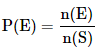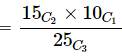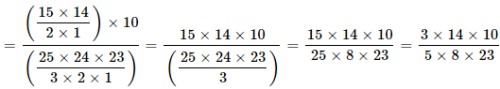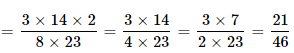Courses

# Test: Probability- 1

## 10 Questions MCQ Test General Aptitude for GATE 2020 | Test: Probability- 1

Description
This mock test of Test: Probability- 1 for Banking Exams helps you for every Banking Exams entrance exam. This contains 10 Multiple Choice Questions for Banking Exams Test: Probability- 1 (mcq) to study with solutions a complete question bank. The solved questions answers in this Test: Probability- 1 quiz give you a good mix of easy questions and tough questions. Banking Exams students definitely take this Test: Probability- 1 exercise for a better result in the exam. You can find other Test: Probability- 1 extra questions, long questions & short questions for Banking Exams on EduRev as well by searching above.
QUESTION: 1

### A bag contains 2 yellow, 3 green and 2 blue balls. Two balls are drawn at random. What is the probability that none of the balls drawn is blue?

Solution: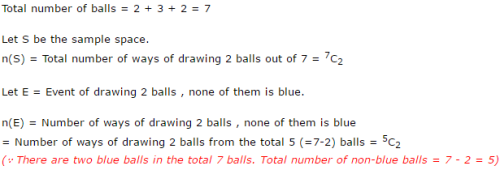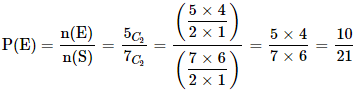QUESTION: 2

### A die is rolled twice. What is the probability of getting a sum equal to 9?

Solution: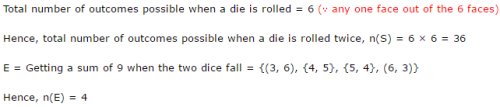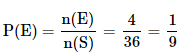QUESTION: 3

### Three coins are tossed. What is the probability of getting at most two tails?

Solution: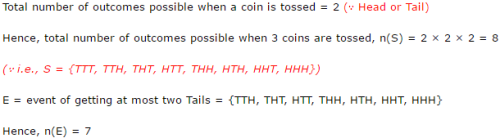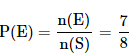QUESTION: 4

When tossing two coins once, what is the probability of heads on both the coins?

Solution: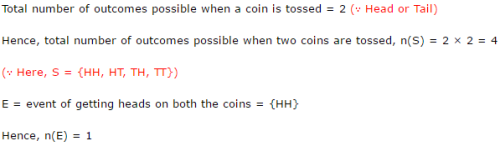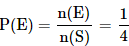QUESTION: 5

What is the probability of getting a number less than 4 when a die is rolled?

Solution: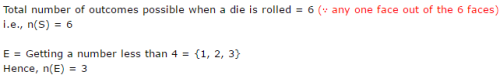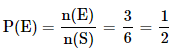QUESTION: 6

A bag contains 4 black, 5 yellow and 6 green balls. Three balls are drawn at random from the bag. What is the probability that all of them are yellow?

Solution: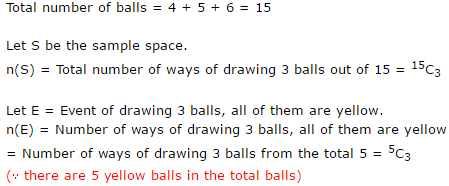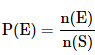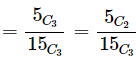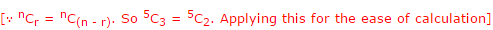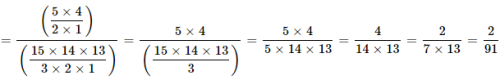QUESTION: 7

One card is randomly drawn from a pack of 52 cards. What is the probability that the card drawn is a face card(Jack, Queen or King)

Solution:

Total number of cards, n(S) = 52

Total number of face cards, n(E) = 12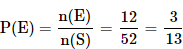QUESTION: 8

A dice is thrown. What is the probability that the number shown in the dice is divisible by 3?

Solution:

Total number of outcomes possible when a die is rolled, n(S) = 6 (? 1 or 2 or 3 or 4 or 5 or 6)

E = Event that the number shown in the dice is divisible by 3 = {3, 6}

Hence, n(E) = 2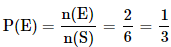QUESTION: 9

John draws a card from a pack of cards. What is the probability that the card drawn is a card of black suit?

Solution:

Total number of cards, n(S) = 52

Total number of black cards, n(E) = 26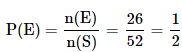QUESTION: 10

There are 15 boys and 10 girls in a class. If three students are selected at random, what is the probability that 1 girl and 2 boys are selected?

Solution:

Let S be the sample space.

n(S) = Total number of ways of selecting 3 students from 25 students = 25C3

Let E = Event of selecting 1 girl and 2 boys

n(E) = Number of ways of selecting 1 girl and 2 boys

15 boys and 10 girls are there in a class.
We need to select 2 boys from 15 boys and 1 girl from 10 girls
Number of ways in which this can be done = 15C2 × 10C1

Hence n(E) = 15C2 × 10C1